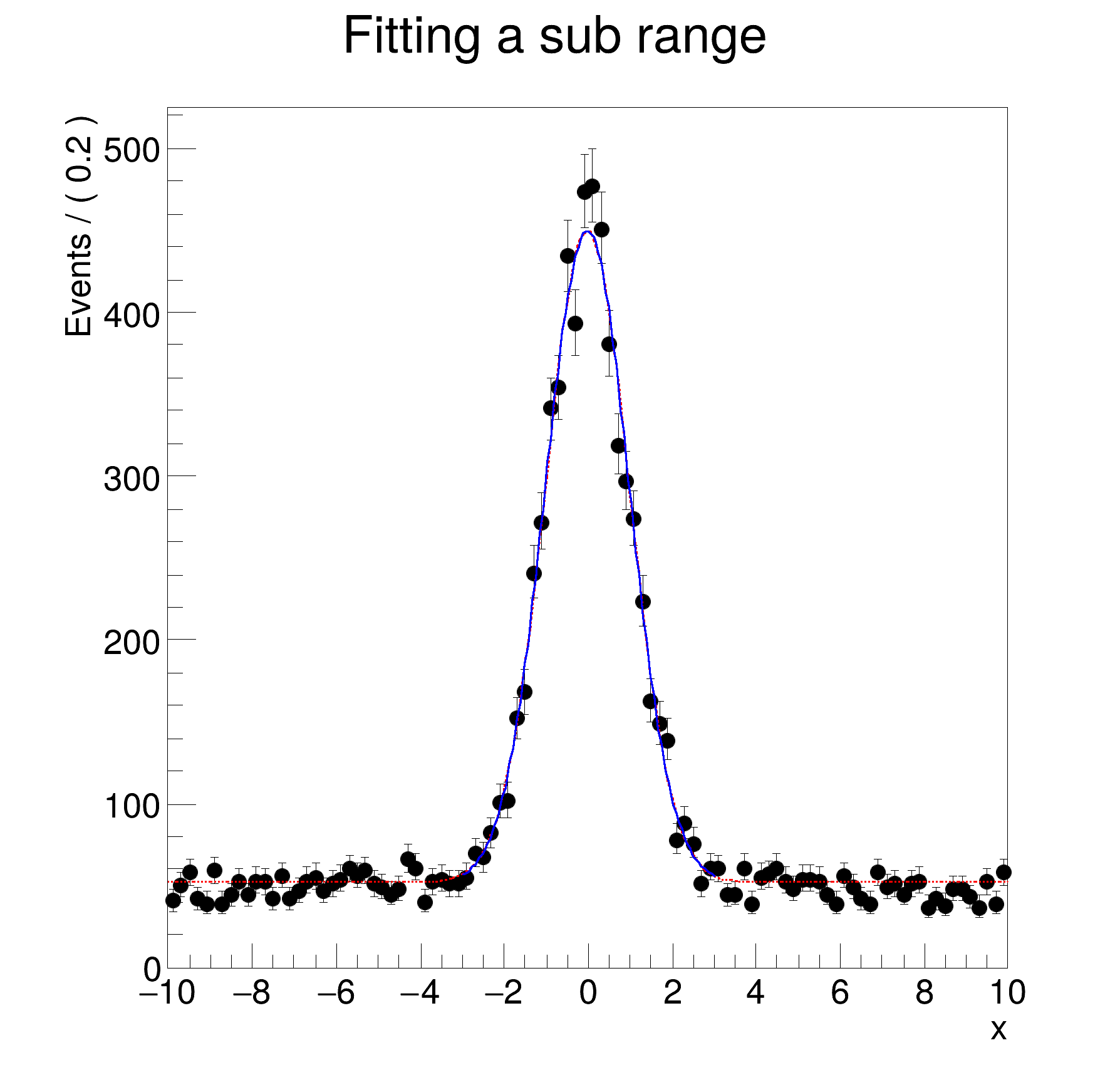ROOT   Reference Guide
Searching...
No Matches
rf203_ranges.py File Reference

## Namespaces

namespace  rf203_ranges

## Detailed DescriptionAddition and convolution: fitting and plotting in sub rangesfrom __future__ import print_function
import ROOT
# Set up model
# ---------------------
# Construct observables x
x = ROOT.RooRealVar("x", "x", -10, 10)
# Construct gaussx(x,mx,1)
mx = ROOT.RooRealVar("mx", "mx", 0, -10, 10)
gx = ROOT.RooGaussian("gx", "gx", x, mx, 1.0)
# px = 1 (flat in x)
px = ROOT.RooPolynomial("px", "px", x)
# model = f*gx + (1-f)px
f = ROOT.RooRealVar("f", "f", 0.0, 1.0)
model = ROOT.RooAddPdf("model", "model", [gx, px], [f])
# Generated 10000 events in (x,y) from pdf model
modelData = model.generate({x}, 10000)
# Fit full range
# ---------------------------
# Fit pdf to all data
r_full = model.fitTo(modelData, Save=True, PrintLevel=-1)
# Fit partial range
# ----------------------------------
# Define "signal" range in x as [-3,3]
x.setRange("signal", -3, 3)
# Fit pdf only to data in "signal" range
r_sig = model.fitTo(modelData, Save=True, Range="signal", PrintLevel=-1)
# Plot/print results
# ---------------------------------------
# Make plot frame in x and add data and fitted model
frame = x.frame(Title="Fitting a sub range")
modelData.plotOn(frame)
model.plotOn(frame, Range="Full", LineColor="r", LineStyle="--") # Add shape in full ranged dashed
model.plotOn(frame) # By default only fitted range is shown
# Print fit results
print("result of fit on all data ")
r_full.Print()
print("result of fit in in signal region (note increased error on signal fraction)")
r_sig.Print()
# Draw frame on canvas
c = ROOT.TCanvas("rf203_ranges", "rf203_ranges", 600, 600)
frame.GetYaxis().SetTitleOffset(1.4)
frame.Draw()
c.SaveAs("rf203_ranges.png")
[#1] INFO:Minimization -- RooAbsMinimizerFcn::setOptimizeConst: activating const optimization
[#1] INFO:Minimization -- The following expressions have been identified as constant and will be precalculated and cached: (px)
[#1] INFO:Minimization -- The following expressions will be evaluated in cache-and-track mode: (gx)
[#1] INFO:Minimization -- RooAbsMinimizerFcn::setOptimizeConst: deactivating const optimization
[#1] INFO:Eval -- RooRealVar::setRange(x) new range named 'signal' created with bounds [-3,3]
[#1] INFO:Eval -- RooRealVar::setRange(x) new range named 'fit_nll_model_modelData' created with bounds [-3,3]
[#1] INFO:Fitting -- RooAbsOptTestStatistic::ctor(nll_model_modelData) constructing test statistic for sub-range named signal
[#1] INFO:Minimization -- RooAbsMinimizerFcn::setOptimizeConst: activating const optimization
[#1] INFO:Minimization -- The following expressions have been identified as constant and will be precalculated and cached: (px)
[#1] INFO:Minimization -- The following expressions will be evaluated in cache-and-track mode: (gx)
[#1] INFO:Minimization -- RooAbsMinimizerFcn::setOptimizeConst: deactivating const optimization
[#1] INFO:Plotting -- RooAbsPdf::plotOn(model) p.d.f was fitted in a subrange and no explicit NormRange() was specified. Plotting / normalising in fit range. To override, do one of the following
- Clear the automatic fit range attribute: <pdf>.removeStringAttribute("fitrange");
- Explicitly specify the plotting range: Range("<rangeName>").
- Explicitly specify where to compute the normalisation: NormRange("<rangeName>").
The default (full) range can be denoted with Range("") / NormRange("").
[#0] ERROR:Plotting -- Range 'Full' not defined for variable 'x'. Ignoring ...
[#1] INFO:Plotting -- RooAbsPdf::plotOn(model) only plotting range 'Full'
[#1] INFO:Plotting -- RooAbsPdf::plotOn(model) p.d.f. curve is normalized using explicit choice of ranges 'fit_nll_model_modelData'
[#1] INFO:Plotting -- RooAbsPdf::plotOn(model) p.d.f was fitted in a subrange and no explicit Range() and NormRange() was specified. Plotting / normalising in fit range. To override, do one of the following
- Clear the automatic fit range attribute: <pdf>.removeStringAttribute("fitrange");
- Explicitly specify the plotting range: Range("<rangeName>").
- Explicitly specify where to compute the normalisation: NormRange("<rangeName>").
The default (full) range can be denoted with Range("") / NormRange("").
[#1] INFO:Plotting -- RooAbsPdf::plotOn(model) only plotting range 'fit_nll_model_modelData'
[#1] INFO:Plotting -- RooAbsPdf::plotOn(model) p.d.f. curve is normalized using explicit choice of ranges 'fit_nll_model_modelData'
RooFitResult: minimized FCN value: 25939.4, estimated distance to minimum: 3.77183e-06
covariance matrix quality: Full, accurate covariance matrix
Status : MINIMIZE=0 HESSE=0
Floating Parameter FinalValue +/- Error
-------------------- --------------------------
f 5.0441e-01 +/- 6.32e-03
mx -2.1605e-02 +/- 1.77e-02
RooFitResult: minimized FCN value: 10339.5, estimated distance to minimum: 0.000279216
covariance matrix quality: Full, accurate covariance matrix
Status : MINIMIZE=0 HESSE=0
Floating Parameter FinalValue +/- Error
-------------------- --------------------------
f 4.8979e-01 +/- 1.62e-02
mx -2.1518e-02 +/- 1.79e-02
result of fit on all data
result of fit in in signal region (note increased error on signal fraction)
Date
February 2018

Definition in file rf203_ranges.py.